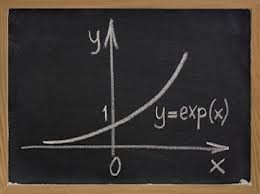Honors: Algebra IICOURSE DESCRIPTIONAlgebra II is a continuation of the algebraic processes begun in Algebra I. This course includes solving equations, inequalities, matrices, graphing, functions, powers, roots, logarithms, complex numbers, polynomials, quadratic relations, sequences, series, probability and statistics. Appropriate technology is integrated into the curriculum.CHAPTERSCh1. – Introduction
Ch2. – Integer Exponents
Ch3. – Scientific Notation
Ch5. – Rational Exponents
Ch6. – Polynomials
Ch7. – Factoring Polynomials
Ch8. – Simplifying Rational Expressions
Ch9. – Multiplying / Dividing Rational Expressions
Ch10. – Adding and Subtracting Rational Expressions
Ch11. – Complex Rational Expressions
Ch12. – Complex Numbers
Ch13. – Test CHAPTERS 2 – 12
Ch14. – Linear Equation in One Variable
Ch15. – Equations with Rational Expressions
Ch16. – Formulas and Applications
Ch17. -  Quadratic Equations
Ch18. – Solving Polynomial Equations by Factoring
Ch19. – Radical Equations Involving Rational Exponents
Ch20. – Equations that are Quadratic in Form
Ch21. – Absolute Value Equations
Ch22. – Linear Inequalities
Ch23. – Quadratic/Rational Inequalities
Ch24. – Test CHAPTERS 14-23
Ch25. –  Slope of a Line
Ch26. – Equations of Lines
Ch27. – Graphing Lines
Ch28. – Parallel and Perpendicular Lines
Ch29. – Circles
Ch30. – Introduction to Functions
Ch31. –  Operations with Functions
Ch32. – Graphs of Functions, Part I
Ch33. – Graphs of Functions, Part II
Ch34. – Test CHAPTERS 15-32

﻿
QQ咨询